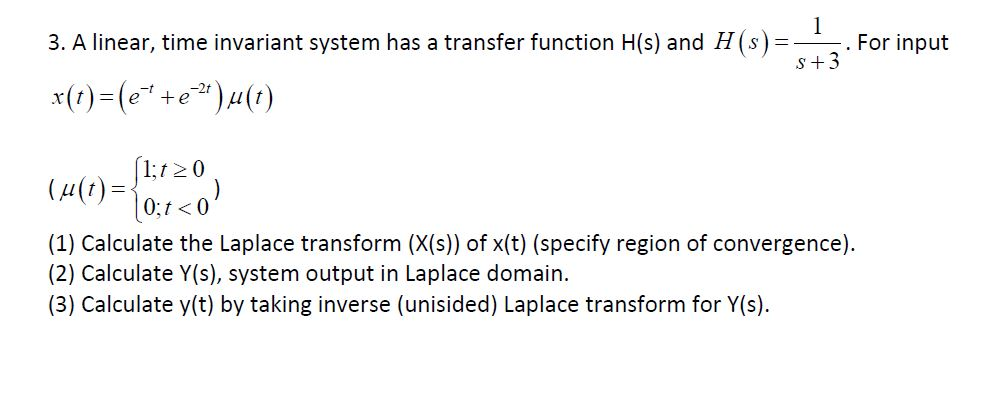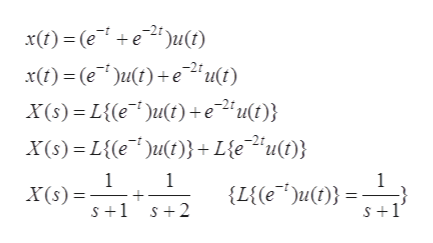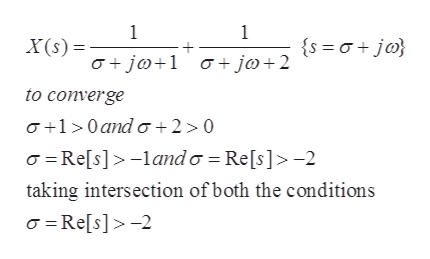# 1. For inputS+33. A linear, time invariant system has a transfer function H(s) and H(s)=xt)(ee)u(t)_1;t0(4()0;t 0(1) Calculate the Laplace transform (X(s)) of x(t) (specify region of convergence)(2) Calculate Y(s), system output in Laplace domain(3) Calculate y(t) by taking inverse (unisided) Laplace transform for Y(s)

Question
5 viewshelp_outlineImage Transcriptionclose1 . For input S+3 3. A linear, time invariant system has a transfer function H(s) and H(s)= xt)(ee)u(t) _ 1;t0 (4() 0;t 0 (1) Calculate the Laplace transform (X(s)) of x(t) (specify region of convergence) (2) Calculate Y(s), system output in Laplace domain (3) Calculate y(t) by taking inverse (unisided) Laplace transform for Y(s) fullscreen
check_circle

Step 1

The input of the given  L.T.I system is:

Step 2

(1)

Calculation of the Laplace transform of the input of the given L.T.I system. The Laplace transform of the given L.T.I system is given by the equation 1, which is calculated as shown below:help_outlineImage Transcriptionclosext)(eeu(€) x(t) (e)u(t)eu(t) X(s) L{e)u(t)+e2*u{t)} X(s) L{e)u(t)} + L{eu(t)} 1 X(s) 1 {L{e*)u)} S+1 2 S+1 fullscreen
Step 3

The area of the convergence of the abov...help_outlineImage Transcriptionclose1 1 X(s) {s ajo jo j@+2 to converge a+10and o+2 > 0 Re[s]lando Re[s]>-2 taking intersection of both the conditions Re[s]-2 fullscreen

### Want to see the full answer?

See Solution

#### Want to see this answer and more?

Solutions are written by subject experts who are available 24/7. Questions are typically answered within 1 hour.*

See Solution
*Response times may vary by subject and question.
Tagged in

### Electrical Engineering